# Monte Carlo methods for improved rendering

https://smerity.com/montelight-cpp/
https://smerity.com/montelight-cpp/code_overview.html

overview
stochastic ray tracing is one of the fundamental algorithms in computer graphics for generating photo-realistic images. we implement an unbiased monte carlo renderer as an experimental testbed 试验台 for evaluating improved sampling strategies. our results show that the improved sampling methods we use for rendering can give comparable image quality over twenty times faster than naive monte carlo methods.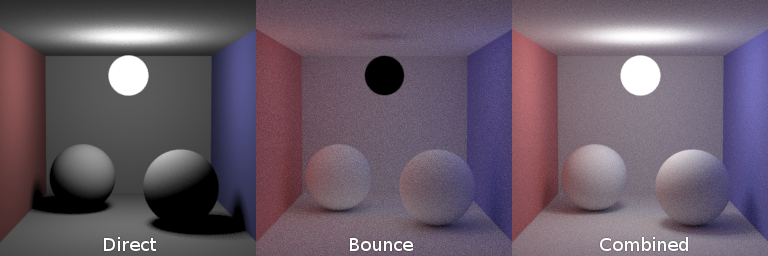approach
to simulate the global reflection of light throughout a scene, we need to consider how light moves around the scene. this is referred to as the light transport equation, described in Kajiya(1986). the basic idea is light is either produced by the object (emission) or reflected onto it from other objects (reflected). for full details, refer to our paper.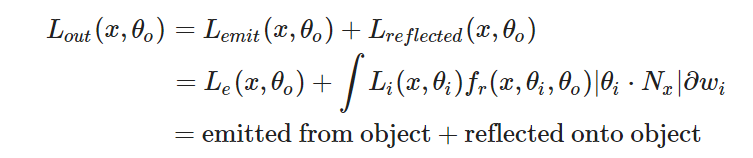the integral for the reflected light is computed over the hemisphere of all possible incoming directions. as this is impossible to compute exactly, it is approximated using monte carlo methods. The primary issue that can occur is when noise is too strong to give reasonable estimations as to the radiance. Whilst the expected value remains accurate, the standard deviation (error) decreases by 1/√n, where nn is the number of samples. To achieve a noise free image requires a large number of samples, especially if the scene is complex.

Importance sampling using BRDF sampling
some materials only reflect light in very specific directions. this is commonly related to the roughness of the material. a mirror, such as the one on the left, needs far less samples than the diffuse surface on the right.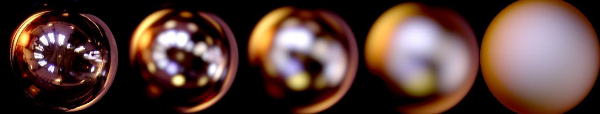this is dictated 决定 by the material’s bidirectional reflectance distribution function (BRDF). this distribution function ditates how much light ends up reflected in a given direction. by sampling directions to test directly from this distribution function (importance sampling), we can avoid taking samples that contribute little to the final image.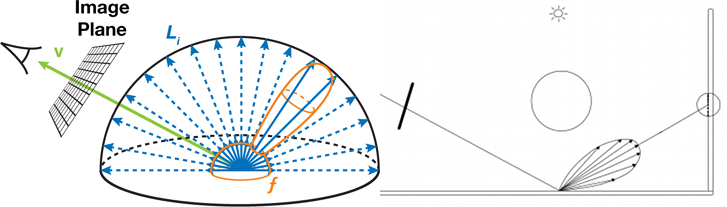Rejection sampling via Russian roulette path termination
bright photons in real life may bounce millions of times, but computing such a long path is intensive and contributes little to the final result. stopping after a finite number of bounces can result in significant bias however.

instead, we introuce Russian roulette path termination, where at each bounce we terminate the light path with probability q. this probability is selected proportional to the overall reflective properties of the material. this means bright objects encourage more bounces whilst同时 dark objects encourage less. as dark objects absorb more light, this means that terminating the path early is unlikely to inrease the noise in the image.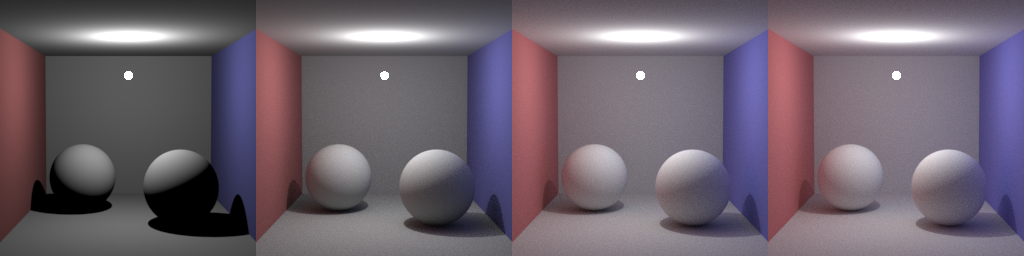The first three images have a maximum allowed light path length of 1, 2, and 3, taking 18 seconds, 31 seconds and 58 seconds respectively. The image on the right uses Russian roulette path termination, taking 83 seconds.

whilst in our scene, extreme bias can primilary be seen in images of maximum allowed light path length of 1 and 2, extreme bias can still be visible in more complex scenes even with max depths of 10 or more.

// ==Montelight==
// Tegan Brennan, Stephen Merity, Taiyo Wilson
#include <cmath>
#include <string>
#include <iomanip>
#include <iostream>
#include <fstream>
#include <sstream>
#include <vector>

#define EPSILON 0.001f

using namespace std;

// Globals
bool EMITTER_SAMPLING = true;

struct Vector {
double x, y, z;
//
Vector(const Vector &o) : x(o.x), y(o.y), z(o.z) {}
Vector(double x_=0, double y_=0, double z_=0) : x(x_), y(y_), z(z_) {}
inline Vector operator+(const Vector &o) const {
return Vector(x + o.x, y + o.y, z + o.z);
}
inline Vector &operator+=(const Vector &rhs) {
x += rhs.x; y += rhs.y; z += rhs.z;
return *this;
}
inline Vector operator-(const Vector &o) const {
return Vector(x - o.x, y - o.y, z - o.z);
}
inline Vector operator*(const Vector &o) const {
return Vector(x * o.x, y * o.y, z * o.z);
}
inline Vector operator/(double o) const {
return Vector(x / o, y / o, z / o);
}
inline Vector operator*(double o) const {
return Vector(x * o, y * o, z * o);
}
inline double dot(const Vector &o) const {
return x * o.x + y * o.y + z * o.z;
}
inline Vector &norm(){
return *this = *this * (1 / sqrt(x * x + y * y + z * z));
}
inline Vector cross(Vector &o){
return Vector(y * o.z - z * o.y, z * o.x - x * o.z, x * o.y - y * o.x);
}
inline double min() {
return fmin(x, fmin(y, z));
}
inline double max() {
return fmax(x, fmax(y, z));
}
inline Vector &abs() {
x = fabs(x); y = fabs(y); z = fabs(z);
return *this;
}
inline Vector &clamp() {
// C++11 lambda function: http://en.cppreference.com/w/cpp/language/lambda
auto clampDouble = [](double x) {
if (x < 0) return 0.0;
if (x > 1) return 1.0;
return x;
};
x = clampDouble(x); y = clampDouble(y); z = clampDouble(z);
return *this;
}
};

struct Ray {
Vector origin, direction;
Ray(const Vector &o_, const Vector &d_) : origin(o_), direction(d_) {}
};

struct Image {
unsigned int width, height;
Vector *pixels, *current;
unsigned int *samples;
std::vector<Vector> *raw_samples;
//
Image(unsigned int w, unsigned int h) : width(w), height(h) {
pixels = new Vector[width * height];
samples = new unsigned int[width * height];
current = new Vector[width * height];
//raw_samples = new std::vector<Vector>[width * height];
}
Vector getPixel(unsigned int x, unsigned int y) {
unsigned int index = (height - y - 1) * width + x;
return current[index];
}
void setPixel(unsigned int x, unsigned int y, const Vector &v) {
unsigned int index = (height - y - 1) * width + x;
pixels[index] += v;
samples[index] += 1;
current[index] = pixels[index] / samples[index];
//raw_samples[index].push_back(v);
}
Vector getSurroundingAverage(int x, int y, int pattern=0) {
unsigned int index = (height - y - 1) * width + x;
Vector avg;
int total;
for (int dy = -1; dy < 2; ++dy) {
for (int dx = -1; dx < 2; ++dx) {
if (pattern == 0 && (dx != 0 && dy != 0)) continue;
if (pattern == 1 && (dx == 0 || dy == 0)) continue;
if (dx == 0 && dy == 0) {
continue;
}
if (x + dx < 0 || x + dx > width - 1) continue;
if (y + dy < 0 || y + dy > height - 1) continue;
index = (height - (y + dy) - 1) * width + (x + dx);
avg += current[index];
total += 1;
}
}
return avg / total;
}
inline double toInt(double x) {
return pow(x, 1 / 2.2f) * 255;
}
void save(std::string filePrefix) {
std::string filename = filePrefix + ".ppm";
std::ofstream f;
f.open(filename.c_str(), std::ofstream::out);
// PPM header: P3 => RGB, width, height, and max RGB value
f << "P3 " << width << " " << height << " " << 255 << std::endl;
// For each pixel, write the space separated RGB values
for (int i=0; i < width * height; i++) {
auto p = pixels[i] / samples[i];
unsigned int r = fmin(255, toInt(p.x)), g = fmin(255, toInt(p.y)), b = fmin(255, toInt(p.z));
f << r << " " << g << " " << b << std::endl;
}
}
void saveHistogram(std::string filePrefix, int maxIters) {
std::string filename = filePrefix + ".ppm";
std::ofstream f;
f.open(filename.c_str(), std::ofstream::out);
// PPM header: P3 => RGB, width, height, and max RGB value
f << "P3 " << width << " " << height << " " << 255 << std::endl;
// For each pixel, write the space separated RGB values
for (int i=0; i < width * height; i++) {
auto p = samples[i] / maxIters;
unsigned int r, g, b;
r= g = b = fmin(255, 255 * p);
f << r << " " << g << " " << b << std::endl;
}
}
~Image() {
delete[] pixels;
delete[] samples;
}
};

struct Shape {
Vector color, emit;
//
Shape(const Vector color_, const Vector emit_) : color(color_), emit(emit_) {}
virtual double intersects(const Ray &r) const { return 0; }
virtual Vector randomPoint() const { return Vector(); }
virtual Vector getNormal(const Vector &p) const { return Vector(); }
};

struct Sphere : Shape {
Vector center;
//
Sphere(const Vector center_, double radius_, const Vector color_, const Vector emit_) :
double intersects(const Ray &r) const {
// Find if, and at what distance, the ray intersects with this object
// Equation follows from solving quadratic equation of (r - c) ^ 2
// http://wiki.cgsociety.org/index.php/Ray_Sphere_Intersection
Vector offset = r.origin - center;
double a = r.direction.dot(r.direction);
double b = 2 * offset.dot(r.direction);
// Find discriminant for use in quadratic equation (b^2 - 4ac)
double disc = b * b - 4 * a * c;
// If the discriminant is negative, there are no real roots
// (ray misses sphere)
if (disc < 0) {
return 0;
}
// The smallest positive root is the closest intersection point
disc = sqrt(disc);
double t = - b - disc;
if (t > EPSILON) {
return t / 2;
}
t = - b + disc;
if (t > EPSILON) {
return t / 2;
}
return 0;
}
Vector randomPoint() const {
// TODO: Improved methods of random point generation as this is not 100% even
// See: https://www.jasondavies.com/maps/random-points/
//
// Get random spherical coordinates on light
double theta = drand48() * M_PI;
double phi = drand48() * 2 * M_PI;
// Convert to Cartesian and scale by radius
double dxr = radius * sin(theta) * cos(phi);
double dyr = radius * sin(theta) * sin(phi);
double dzr = radius * cos(theta);
return Vector(center.x + dxr, center.y + dyr, center.z + dzr);
}
Vector getNormal(const Vector &p) const {
// Point must have collided with surface of sphere which is at radius
// Normalize the normal by using radius instead of a sqrt call
return (p - center) / radius;
}
};

// Set up our testing scenes
// They're all Cornell box inspired: http://graphics.ucsd.edu/~henrik/images/cbox.html
/////////////////////////
// Scene format: Sphere(position, radius, color, emission)
/////////////////////////
std::vector<Shape *> simpleScene = {
// Left sphere
new Sphere(Vector(1e5+1,40.8,81.6), 1e5f, Vector(.75,.25,.25), Vector()),
// Right sphere
new Sphere(Vector(-1e5+99,40.8,81.6), 1e5f, Vector(.25,.25,.75), Vector()),
// Back sphere
new Sphere(Vector(50,40.8, 1e5), 1e5f, Vector(.75,.75,.75), Vector()),
// Floor sphere
new Sphere(Vector(50, 1e5, 81.6), 1e5f, Vector(.75,.75,.75), Vector()),
// Roof sphere
new Sphere(Vector(50,-1e5+81.6,81.6), 1e5f, Vector(.75,.75,.75), Vector()),
new Sphere(Vector(27,16.5,47), 16.5f, Vector(1,1,1) * 0.799, Vector()),
new Sphere(Vector(73,16.5,78), 16.5f, Vector(1,1,1) * 0.799, Vector()),
// Light source
//new Sphere(Vector(50,681.6-.27,81.6), 600, Vector(1,1,1) * 0.5, Vector(12,12,12))
new Sphere(Vector(50,65.1,81.6), 8.5, Vector(), Vector(4,4,4) * 100) // Small = 1.5, Large = 8.5
};
/////////////////////////
std::vector<Shape *> complexScene = {
new Sphere(Vector(1e5+1,40.8,81.6), 1e5f, Vector(.75,.25,.25), Vector()), // Left
new Sphere(Vector(-1e5+99,40.8,81.6), 1e5f, Vector(.25,.25,.75), Vector()), // Right
new Sphere(Vector(50,40.8, 1e5), 1e5f, Vector(.75,.75,.75), Vector()), // Back
new Sphere(Vector(50, 1e5, 81.6), 1e5f, Vector(.75,.75,.75), Vector()), //Bottom
new Sphere(Vector(50,-1e5+81.6,81.6), 1e5f, Vector(.75,.75,.75), Vector()), // Top
new Sphere(Vector(20,16.5,40), 16.5f, Vector(1,1,1) * 0.799, Vector()),
new Sphere(Vector(50,16.5,80), 16.5f, Vector(1,1,1) * 0.799, Vector()),
new Sphere(Vector(75,16.5,120), 16.5f, Vector(1,1,1) * 0.799, Vector()),
new Sphere(Vector(50,65.1,40), 1.5, Vector(), Vector(4,4,4) * 100), // Light
new Sphere(Vector(50,65.1,120), 1.5, Vector(), Vector(4,4,4) * 100), // Light
};
//

struct Tracer {
std::vector<Shape *> scene;
//
Tracer(const std::vector<Shape *> &scene_) : scene(scene_) {}
std::pair<Shape *, double> getIntersection(const Ray &r) const {
Shape *hitObj = NULL;
double closest = 1e20f;
for (Shape *obj : scene) {
double distToHit = obj->intersects(r);
if (distToHit > 0 && distToHit < closest) {
hitObj = obj;
closest = distToHit;
}
}
return std::make_pair(hitObj, closest);
}
Vector getRadiance(const Ray &r, int depth) {
// Work out what (if anything) was hit
auto result = getIntersection(r);
Shape *hitObj = result.first;
// Russian Roulette sampling based on reflectance of material
double U = drand48();
if (depth > 4 && (depth > 20 || U > hitObj->color.max())) {
return Vector();
}
Vector hitPos = r.origin + r.direction * result.second;
Vector norm = hitObj->getNormal(hitPos);
// Orient the normal according to how the ray struck the object
if (norm.dot(r.direction) > 0) {
norm = norm * -1;
}
// Work out the contribution from directly sampling the emitters
Vector lightSampling;
if (EMITTER_SAMPLING) {
for (Shape *light : scene) {
// Skip any objects that don't emit light
if (light->emit.max() == 0) {
continue;
}
Vector lightPos = light->randomPoint();
Vector lightDirection = (lightPos - hitPos).norm();
Ray rayToLight = Ray(hitPos, lightDirection);
auto lightHit = getIntersection(rayToLight);
if (light == lightHit.first) {
double wi = lightDirection.dot(norm);
if (wi > 0) {
double omega = 2*M_PI*(1-cos_a_max);
lightSampling += light->emit * wi * omega * M_1_PI;
}
}
}
}
// Work out contribution from reflected light
// Diffuse reflection condition:
// Create orthogonal coordinate system defined by (x=u, y=v, z=norm)
double angle = 2 * M_PI * drand48();
double dist_cen = sqrt(drand48());
Vector u;
if (fabs(norm.x) > 0.1) {
u = Vector(0, 1, 0);
} else {
u = Vector(1, 0, 0);
}
u = u.cross(norm).norm();
Vector v = norm.cross(u);
// Direction of reflection
Vector d = (u * cos(angle) * dist_cen + v * sin(angle) * dist_cen + norm * sqrt(1 - dist_cen * dist_cen)).norm();

// Recurse
Vector reflected = getRadiance(Ray(hitPos, d), depth + 1);
//
if (!EMITTER_SAMPLING || depth == 0) {
return hitObj->emit + hitObj->color * lightSampling + hitObj->color * reflected;
}
return hitObj->color * lightSampling + hitObj->color * reflected;
}
};

int main(int argc, const char *argv[]) {
/////////////////////////
// Variables to modify the process or the images
EMITTER_SAMPLING = true;
int w = 256, h = 256;
int SNAPSHOT_INTERVAL = 10;
unsigned int SAMPLES = 50;
bool FOCUS_EFFECT = false;
double FOCAL_LENGTH = 35;
double APERTURE_FACTOR = 1; // ratio of original/new aperture (>1: smaller view angle, <1: larger view angle)
// Initialize the image
Image img(w, h);
/////////////////////////
// Set which scene should be raytraced
auto &scene = complexScene;
Tracer tracer = Tracer(scene);
/////////////////////////
// Set up the camera
double aperture = 0.5135 / APERTURE_FACTOR;
Vector cx = Vector((w * aperture) / h, 0, 0);
Vector dir_norm = Vector(0, -0.042612, -1).norm();
double L = 140;
double L_new = APERTURE_FACTOR * L;
double L_diff = L - L_new;
Vector cam_shift = dir_norm * (L_diff);
if (L_diff < 0){
cam_shift = cam_shift * 1.5;
}
L = L_new;
Ray camera = Ray(Vector(50, 52, 295.6) + cam_shift, dir_norm);
// Cross product gets the vector perpendicular to cx and the "gaze" direction
Vector cy = (cx.cross(camera.direction)).norm() * aperture;
/////////////////////////
// Take a set number of samples per pixel
for (int sample = 0; sample < SAMPLES; ++sample) {
std::cout << "Taking sample " << sample << "\r" << std::flush;
if (sample && sample % SNAPSHOT_INTERVAL == 0) {
std::ostringstream fn;
fn << std::setfill('0') << std::setw(5) << sample;
img.save("temp/render_" + fn.str());
}
// For each pixel, sample a ray in that direction
for (int y = 0; y < h; ++y)
{
for (int x = 0; x < w; ++x)
{
/*
Vector target = img.getPixel(x, y);
double A = (target - img.getSurroundingAverage(x, y, sample % 2)).abs().max() / (100 / 255.0);
if (sample > 10 && drand48() > A) {
continue;
}
++updated;
*/
// Jitter pixel randomly in dx and dy according to the tent filter
double Ux = 2 * drand48();
double Uy = 2 * drand48();
double dx;
if (Ux < 1) {
dx = sqrt(Ux) - 1;
} else {
dx = 1 - sqrt(2 - Ux);
}
double dy;
if (Uy < 1) {
dy = sqrt(Uy) - 1;
} else {
dy = 1 - sqrt(2 - Uy);
}
// Calculate the direction of the camera ray
Vector d = (cx * (((x+dx) / float(w)) - 0.5)) + (cy * (((y+dy) / float(h)) - 0.5)) + camera.direction;
Ray ray = Ray(camera.origin + d * 140, d.norm());
// If we're actually using depth of field, we need to modify the camera ray to account for that
if (FOCUS_EFFECT) {
// Calculate the focal point
Vector fp = (camera.origin + d * L) + d.norm() * FOCAL_LENGTH;
// Get a pixel point and new ray direction to calculate where the rays should intersect
Vector del_x = (cx * dx * L / float(w));
Vector del_y = (cy * dy * L / float(h));
Vector point = camera.origin + d * L;
point = point + del_x + del_y;
d = (fp - point).norm();
ray = Ray(camera.origin + d * L, d.norm());
}
// Retrieve the radiance of the given hit location (i.e. brightness of the pixel)
// Clamp the radiance so it is between 0 and 1
// If we don't do this, antialiasing doesn't work properly on bright lights
// Add result of sample to image
}
}
}
// Save the resulting raytraced image
img.save("render");
return 0;
}



std::cout << "Taking sample " << sample << "\r" << std::flush;
if (sample && sample % SNAPSHOT_INTERVAL == 0)
{
std::ostringstream fn;
fn << std::setfill('0') << std::setw(5) << sample;
img.save("temp/render_" + fn.str());
}


tracer
the tracer class is responsible for handling all ray and light path calculations in the scene. this is broken up into the getIntersection and getRadiance function. the getIntersection function takes a ray and returns the distance to the first object the ray hits, or NULL if no object is hit.

https://www.scratchapixel.com/lessons/3d-basic-rendering/minimal-ray-tracer-rendering-simple-shapes/ray-sphere-intersection

std::pair<Shape *, double> getIntersection(const Ray &r) const
{
Shape *hitObj = NULL;
double closest = 1e20f;
for (Shape *obj : scene)
{
double distToHit = obj->intersects(r);
if (distToHit > 0 && distToHit < closest)
{
hitObj = obj;
closest = distToHit;
}
}
return std::make_pair(hitObj, closest);
}


the core of the raytracer is the getRadiance function, which returns a sample of the amout of lighting coming in from a set direction when given a ray. this is where the logic for BRDF sampling, explicit light sampling, and Russian roulette path termination occur.

the rejection sampling for Russian roulette path termination occurs when we test u>hitObj->color.max(). we sample a random number U∈[0,1] and take the maximum color component of the object, where the color components are confined to [0,1]. if the object is dark and absorbs the majority of light that reaches it, the probability for path termination is high. conversely, if the object reflects all of any of the color components, it has a zero probability of terminating the path at that point. if the path is terminated, we return zero light contribution (as Vector() defaults to all zeroes, or black).

Vector getRadiance(const Ray &r, int depth) {
// Work out what (if anything) was hit
auto result = getIntersection(r);
Shape *hitObj = result.first;
// Russian Roulette sampling based on reflectance of material
double U = drand48();
if (depth > 4 && (depth > 20 || U > hitObj->color.max())) {
return Vector();
}
Vector hitPos = r.origin + r.direction * result.second;
Vector norm = hitObj->getNormal(hitPos);
// Orient the normal according to how the ray struck the object
if (norm.dot(r.direction) > 0) {
norm = norm * -1;
}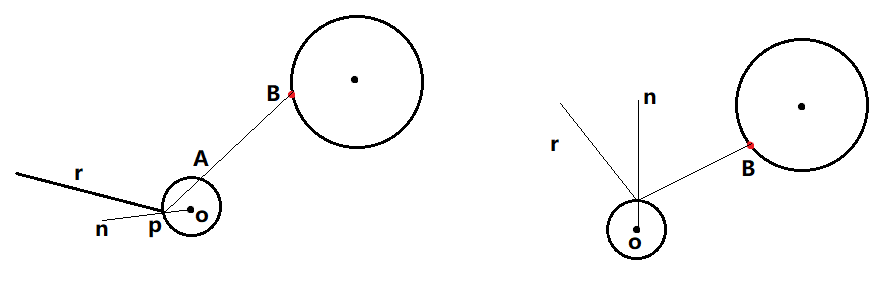for explicit light sampling, we first check if it is enabled. if it is, we sample a random point from each light in the scene and check if it illustrates the current hit surface. 这句话很重要，遍历当前场景中所有的发光的物体，让其照亮当前的处理的点。
to work out the direction to the light, we use the trick described earlier,
(lightPos - hitPos).norm() .
with that, we can describe a ray from the hit surface (point) towards the light source via
Ray(hitPost, lightDirection).
we then check if the given ray immediately hits the light source. if it hits any other object, we know the object is in shadow, and receives no direct light contribution from that point. this is well visualized by the diagram below.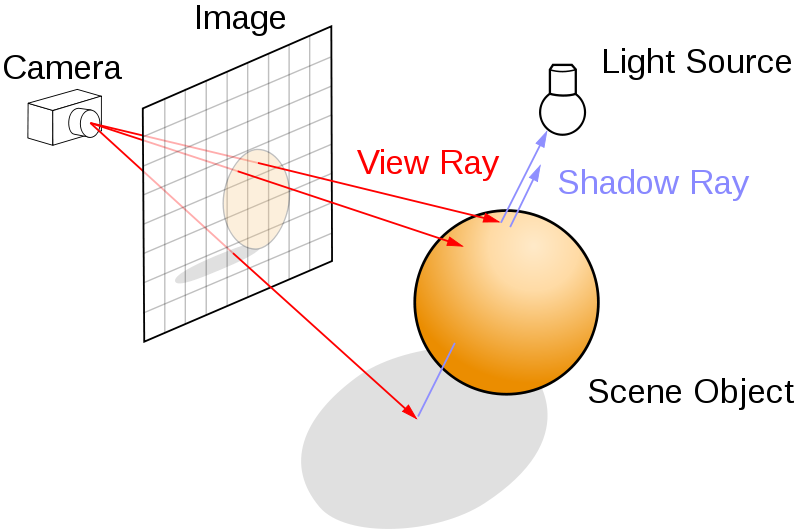the rays that hit the top of the sphere are illuminated by the light source as there is nothing between it and the light. the ray that hits underneath the sphere first hits the sphere when travelling towards the light source, so we know it is in shadow.

if the hit surface is illuminated by the random point on the light, we calcualte the angle towards it using the other vector trick mentioned earlier, lightDirection.dot(norm).
this calcualtes the angle between the normal of the hit surface and the light source, cosθ. then we employ a simple BRDF to approximate how much of the light would be sent from the light source to this specific hit surface. for more details about the BRDF, refer to An Introduction to BRDF-Base Lighting or our paper.

 // Work out the contribution from directly sampling the emitters
Vector lightSampling;
if (EMITTER_SAMPLING) {
for (Shape *light : scene) {
// Skip any objects that don't emit light
if (light->emit.max() == 0) {
continue;
}
Vector lightPos = light->randomPoint();
Vector lightDirection = (lightPos - hitPos).norm();
Ray rayToLight = Ray(hitPos, lightDirection);
auto lightHit = getIntersection(rayToLight);
if (light == lightHit.first) {
double wi = lightDirection.dot(norm);
if (wi > 0) {
double omega = 2*M_PI*(1-cos_a_max);
lightSampling += light->emit * wi * omega * M_1_PI;
}
}
}
}


finally, we work out the amout of light that would be reflected onto this location from another surface in the scene. to do this, we must select a random direction to travel. this random direction is selected again according to the material’s BRDF in order to minimize wasted rays.// Work out contribution from reflected light
// Diffuse reflection condition:
// Create orthogonal coordinate system defined by (x=u, y=v, z=norm)
double angle = 2 * M_PI * drand48();
double dist_cen = sqrt(drand48());
Vector u;
if (fabs(norm.x) > 0.1) {
u = Vector(0, 1, 0);
} else {
u = Vector(1, 0, 0);
}
u = u.cross(norm).norm();
Vector v = norm.cross(u);
// Direction of reflection
Vector d = (u * cos(angle) * dist_cen + v * sin(angle) * dist_cen + norm * sqrt(1 - dist_cen * dist_cen)).norm();


finally, we launch the ray in the given direction and record how much light is sent back. depending on whether we are using explicit light sampling or not, we have two different paths. emitter sampling can not consider light emitted from the objects it hits due to double counting. for full details, refere to our paper.

// Recurse
Vector reflected = getRadiance(Ray(hitPos, d), depth + 1);
//
if (!EMITTER_SAMPLING || depth == 0) {
return hitObj->emit + hitObj->color * lightSampling + hitObj->color * reflected;
}
return hitObj->color * lightSampling + hitObj->color * reflected;
}
};


©️2019 CSDN 皮肤主题: 创作都市 设计师: CSDN官方博客